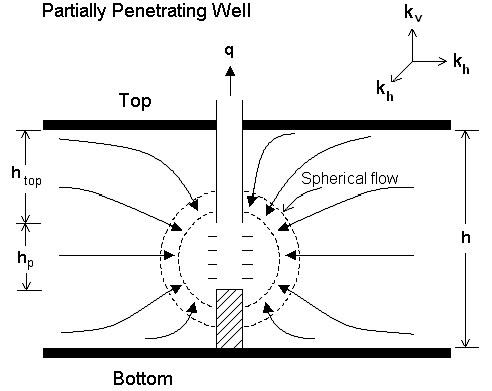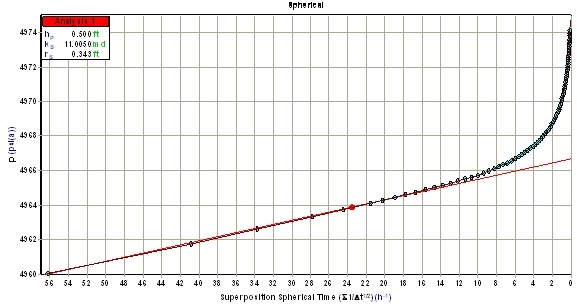# Spherical Flow Analysis

When a well is partially penetrating, or partially completed into the formation, the well is connected to the producing interval (pay thickness) on one fraction of the zone only. As the contact area between the reservoir and the well is reduced, some fluid has to travel further through the formation to get produced through the wellbore (see the figure below). Thus, an extra pressure drop is created around the wellbore for a given production rate, which can be characterized as a skin factor due to partial penetration (spp).Spherical flow analysis can be used prior to the start of the infinite-acting radial flow regime at very early times. To apply the spherical flow model in the case of production through a cylindrical wellbore, an equivalent spherical wellbore radius (rs) needs to be applied, which acts as the wellbore radius (rw) for all calculations involving spherical flow. This is calculated as follows:

For hp / 2 <= rw:For hp / 2 > rw:During the spherical flow regime, the pressure drawdown occurring with time can be expressed as:Spherical permeability is defined as:The above equation can be manipulated to show the expression for spherical permeability as:Where:Spherical flow data forms a straight line when placed on a semi-log plot of pressure versus an inverse square root time function (p vs. 1 / sqrt (time function)) for a buildup as shown below. m is defined as the slope of this line. Note that to perform a spherical flow analysis, the value of hp must be specified to calculate the values of ks and rs.## References

1. "Pressure Buildup Equations for Spherical Flow Regime Problems", W.E. Culham, SPEJ (December 1974) 545 - 555.

2. "Theoretical Analysis of Pressure Phenomena Associated with the Wireline Formation Tester", J.H. Moran and E.E. Finklea, JPT (August 1962) 899 - 908.